Collision between two or more particles is the interaction for a short interval of time in which they apply relatively strong forces on each other.

We shall study the collision of two masses in an idealized form. Consider two masses m1 and m2 The particle m1 is moving with speed v1i , the subscript ‘i’ implying initially. We can consider m2 to be at rest. No loss of generality is involved in making such a selection. In this situation, the mass m1 collides with the stationary mass m2 and this is depicted in Fig.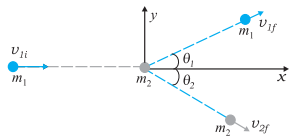Fig.1. The collision of mass m1 , with a stationary mass m2.

The masses m1 and m2 fly-off in different directions. We shall see that there are relationships, which connect the masses, the velocities, and the angles.

In all collisions the total linear momentum is conserved; the initial momentum of the system is equal to the final momentum of the system.

### There are two types of collisions:

• Elastic Collisions
• Inelastic Collisions

# Elastic Collisions

If the kinetic energy of the system is conserved during a collision, it is called an elastic collision. (i.e) The total kinetic energy before the collision and after collision remains unchanged. The collision between subatomic particles is generally elastic. The collision between two steel or glass balls is nearly elastic. In the elastic collision, the linear momentum and kinetic energy of the system are conserved.

## Elastic collision in one dimension:

If the two bodies after collision move in a straight line, the collision is said to be of one dimension.

Consider two bodies A and B of masses m1 and m2 moving along the same straight line in the same direction with velocities u1 and u2 respectively as shown in Fig.. Let us assume that u1 is greater than u2. The bodies A and B suffer a head on collision when they strike and continue to move along the same straight line with velocities v1 and v2 respectively.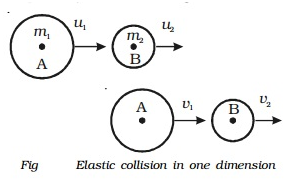From the law of conservation of linear momentum,

Total momentum before collision = Total momentum after collision

m1u1 + m2u2 = m1v1 + m1v1 ——- (1)

Since the kinetic energy of the bodies is also conserved during the collision

Total kinetic energy before collision = Total kinetic energy after collision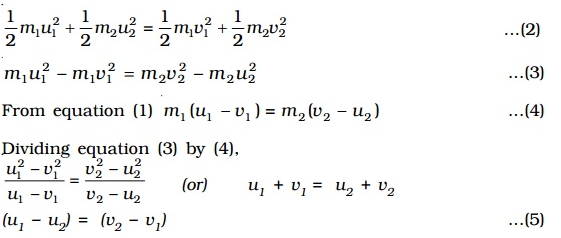Equation 5 shows that in an elastic one-dimensional collision, the relative velocity with which the two bodies approach each other before the collision is equal to the relative velocity with which they recede from each other after the collision.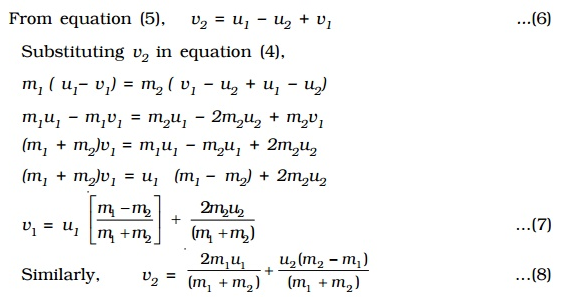### Special cases for elastic collision:

Case 1: If the masses of colliding bodies are equal, i.e. m1 = m2 , v1 = u2 and v2 = u1 .

After a head on elastic collision, the velocities of the colliding bodies are mutually interchanged.

Case 2: If the particle B is initially at rest, (i.e.) u2 = 0 then

If the initial velocities and final velocities of both the bodies are along the same straight line, then it is called a one-dimensional collision, or a head-on collision. In the case of small spherical bodies, this is possible if the direction of travel of body 1 passes through the center of body 2 which is at rest. In general, the collision is two dimensional, where the initial velocities and the final velocities lie in a plane.

## Collision in Two Dimensions:

Linear momentum is conserved in such a collision. Since momentum is a vector this implies three equations for the three directions {x, y, z}. Consider the plane determined by the final velocity directions of m1 and m2 and choose it to be the x-y plane. The conservation of the z-component of the linear momentum implies that the entire collision is in the x-y plane. The x– and y-component equations are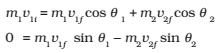One knows (m1 , m2 , v1i ) in most situations. There are thus four unknowns ( v1f , v2f , θ1 and θ2 ), and only
two equations. If θ1 = θ2 = 0,

We regain this equation eq. (1) for one dimensional equation.

If, further the collision is elastic,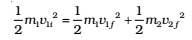We obtain an additional equation. That still leaves us one equation short.